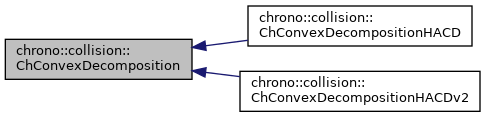chrono::collision::ChConvexDecomposition Class Referenceabstract

Description

Base interface class for convex decomposition.

There are some inherited classes that can be instanced to perform different algorithms of decomposition.

#include <ChCConvexDecomposition.h>

Inheritance diagram for chrono::collision::ChConvexDecomposition:[legend]

Public Member Functions

ChConvexDecomposition ()
Basic constructor.

virtual ~ChConvexDecomposition ()
Destructor.

virtual void Reset (void)=0
Reset the input mesh data.

virtual bool AddTriangle (const ChVector<> &v1, const ChVector<> &v2, const ChVector<> &v3)=0
Add a triangle, by passing three points for vertexes. More...

virtual bool AddTriangle (const geometry::ChTriangle &t1)
Add a triangle, by passing a ChTriangle object (that will be copied, not referenced). More...

virtual bool AddTriangleMesh (const geometry::ChTriangleMesh &tm)
Add a triangle mesh, by passing an entire ChTriangleMesh object. More...

virtual int ComputeConvexDecomposition ()=0
Perform the convex decomposition.

virtual unsigned int GetHullCount ()=0
Get the number of computed hulls after the convex decomposition.

virtual bool GetConvexHullResult (unsigned int hullIndex, geometry::ChTriangleMesh &convextrimesh)=0
Get the n-th computed convex hull, by filling a ChTriangleMesh object that is passed as a parameter. More...

virtual bool GetConvexHullResult (unsigned int hullIndex, std::vector< ChVector< double > > &convexhull)=0
Get the n-th computed convex hull, by filling a vector of points of the vertexes of the n-th hull that is passed as a parameter. More...

virtual bool WriteConvexHullsAsChullsFile (ChStreamOutAscii &mstream)
Write the convex decomposition to a ".chulls" file, where each hull is a sequence of x y z coords. More...

virtual void WriteConvexHullsAsWavefrontObj (ChStreamOutAscii &mstream)=0
Save the computed convex hulls as a Wavefront file using the '.obj' fileformat, with each hull as a separate group. More...

Member Function Documentation

 virtual bool chrono::collision::ChConvexDecomposition::AddTriangle ( const ChVector<> & v1, const ChVector<> & v2, const ChVector<> & v3 )
pure virtual

Add a triangle, by passing three points for vertexes.

Note: the vertexes must be properly ordered (oriented triangle, normal pointing outside)

 bool chrono::collision::ChConvexDecomposition::AddTriangle ( const geometry::ChTriangle & t1 )
virtual

Add a triangle, by passing a ChTriangle object (that will be copied, not referenced).

Note: the vertexes must be properly ordered (oriented triangle, normal pointing outside)

 bool chrono::collision::ChConvexDecomposition::AddTriangleMesh ( const geometry::ChTriangleMesh & tm )
virtual

Add a triangle mesh, by passing an entire ChTriangleMesh object.

Note: the triangles must define closed volumes (holes, gaps in edges, etc. may trouble the decomposition)

 virtual bool chrono::collision::ChConvexDecomposition::GetConvexHullResult ( unsigned int hullIndex, geometry::ChTriangleMesh & convextrimesh )
pure virtual

Get the n-th computed convex hull, by filling a ChTriangleMesh object that is passed as a parameter.

 virtual bool chrono::collision::ChConvexDecomposition::GetConvexHullResult ( unsigned int hullIndex, std::vector< ChVector< double > > & convexhull )
pure virtual

Get the n-th computed convex hull, by filling a vector of points of the vertexes of the n-th hull that is passed as a parameter.

 bool chrono::collision::ChConvexDecomposition::WriteConvexHullsAsChullsFile ( ChStreamOutAscii & mstream )
virtual

Write the convex decomposition to a ".chulls" file, where each hull is a sequence of x y z coords.

Can throw exceptions.

 virtual void chrono::collision::ChConvexDecomposition::WriteConvexHullsAsWavefrontObj ( ChStreamOutAscii & mstream )
pure virtual

Save the computed convex hulls as a Wavefront file using the '.obj' fileformat, with each hull as a separate group.

May throw exceptions if file locked etc.